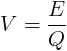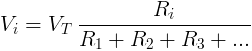# Electrical Voltage

Electrical voltage is defined as electric potential difference between two points of an electric field.

Using water pipe analogy, we can visualize the voltage as height difference that makes the water flow down.

V = φ2 - φ1

V is the voltage between point 2 and 1 in volts (V).

φ2 is the electric potential at point #2 in volts (V).

φ1 is the electric potential at point #1 in volts (V).

In an electrical circuit, the electrical voltage V in volts (V) is equal to the energy consumption E in joules (J)

divided by the electric charge Q in coulombs (C).V is the voltage measured in volts (V)

E is the energy measured in joules (J)

Q is the electric charge measured in coulombs (C)

## Voltage in series

The total voltage of several voltage sources or voltage drops in series is their sum.

VT = V1 + V2 + V3 +...

VT - the equivalent voltage source or voltage drop in volts (V).

V1 - voltage source or voltage drop in volts (V).

V2 - voltage source or voltage drop in volts (V).

V3 - voltage source or voltage drop in volts (V).

## Voltage in parallel

Voltage sources or voltage drops in parallel have equal voltage.

VT = V1 = V2 = V3 =...

VT - the equivalent voltage source or voltage drop in volts (V).

V1 - voltage source or voltage drop in volts (V).

V2 - voltage source or voltage drop in volts (V).

V3 - voltage source or voltage drop in volts (V).

## Voltage divider

For electrical circuit with resistors (or other impedance) in series, the voltage drop Vi on resistor Ri is:## Kirchhoff's voltage law (KVL)

The sum of voltage drops at a current loop is zero.

Vk = 0

## DC circuit

Direct current (DC) is generated by a constant voltage source like a battery or DC voltage source.

The voltage drop on a resistor can be calculated from the resistor's resistance and the resistor's current, using Ohm's law:

##### Voltage calculation with Ohm's law

VR = IR × R

VR - voltage drop on the resistor measured in volts (V)

IR - current flow through the resistor measured in amperes (A)

R - resistance of the resistor measured in ohms (Ω)

## AC circuit

Alternating current is generated by a sinusoidal voltage source.

##### Ohm's law

VZ = IZ × Z

VZ - voltage drop on the load measured in volts (V)

IZ - current flow through the load measured in amperes (A)

Z - impedance of the load measured in ohms (Ω)

##### Momentary voltage

v(t) = Vmax × sin(ωt)

v(t) - voltage at time t, measured in volts (V).

Vmax - maximal voltage (=amplitude of sine), measured in volts (V).

t        - time, measured in seconds (s).

##### RMS (effective) voltage

VrmsVeff  =  Vmax / √2 ≈ 0.707 Vmax

Vrms -  RMS voltage, measured in volts (V).

Vmax - maximal voltage (=amplitude of sine), measured in volts (V).

Vp-p = 2Vmax

## Voltage drop

Voltage drop is the drop of electrical potential or potential difference on the load in an electrical circuit.

## Voltage Measurement

Electrical voltage is measured with Voltmeter. The Voltmeter is connected in parallel to the measured component or circuit.

The voltmeter has very high resistance, so it almost does not affect the measured circuit.

## Voltage by Country

AC voltage supply may vary for each country.

European countries use 230V while north America countries use 120V.

Country Voltage

[Volts]

Frequency

[Hertz]

Australia 230V 50Hz
Brazil 110V 60Hz
China 220V 50Hz
France 230V 50Hz
Germany 230V 50Hz
India 230V 50Hz
Ireland 230V 50Hz
Israel 230V 50Hz
Italy 230V 50Hz
Japan 100V 50/60Hz
New Zealand 230V 50Hz
Philippines 220V 60Hz
Russia 220V 50Hz
South Africa 220V 50Hz
Thailand 220V 50Hz
UK 230V 50Hz
USA 120V 60Hz

Electrical current